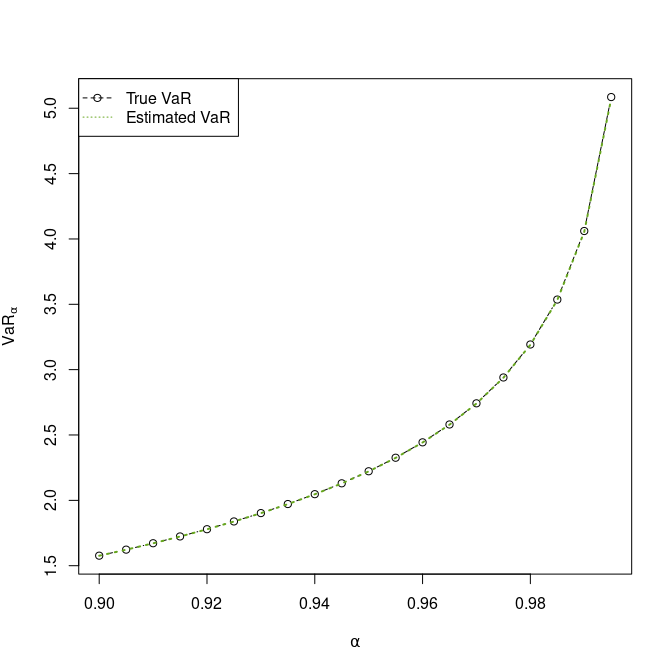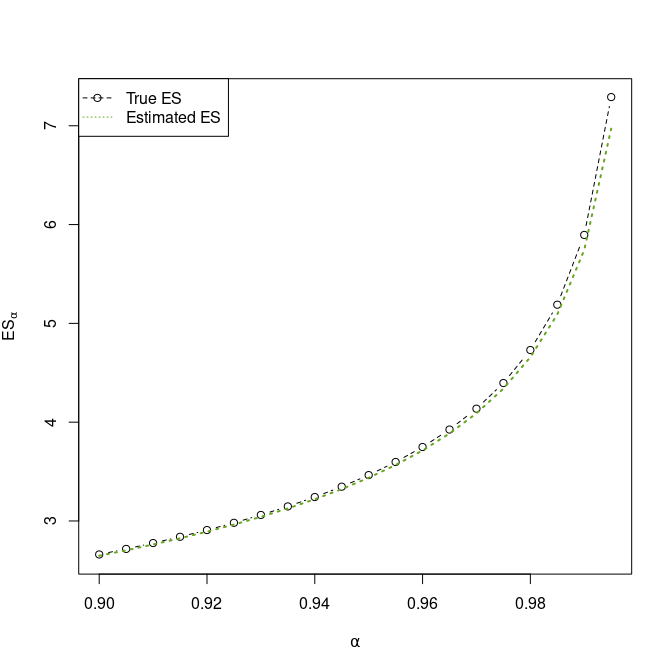# Estimating risk measures for normal variance mixture distributions

#### 2022-04-26

library(nvmix)
library(RColorBrewer)
doPDF <- FALSE

## Introduction

A random vector $$\mathbf{X}=(X_1,\dots,X_d)$$ follows a normal variance mixture, in notation $$\mathbf{X}\sim \operatorname{NVM}_d(\mathbf{\mu},\Sigma,F_W)$$, if, in distribution, $\mathbf{X} = \mathbf{\mu}+\sqrt{W}A\mathbf{Z},$ where $$\mathbf{\mu}\in\mathbb{R}^d$$ denotes the location (vector), $$\Sigma=AA^\top$$ for $$A\in\mathbb{R}^{d\times k}$$ denotes the scale (matrix) (a covariance matrix), and the mixture variable $$W\sim F_W$$ is a non-negative random variable independent of $$\mathbf{Z}\sim\operatorname{N}_k(\mathbf{0},I_k)$$ (where $$I_k\in\mathbb{R}^{k\times k}$$ denotes the identity matrix). Both the Student’s $$t$$ distribution with degrees of freedom parameter $$\nu>0$$ and the normal distribution are normal variance mixtures; in the former case, $$W\sim\operatorname{IG}(\nu/2, \nu/2)$$ (inverse gamma) and in the latter case $$W$$ is almost surely constant (taken as $$1$$ so that $$\Sigma$$ is the covariance matrix of $$\mathbf{X}$$ in this case).

It follows readily from the stochastic representation that linear combinations of multivariate normal variance mixtures are univariate normal variance mixtures. Let $$\mathbf{a}\in\mathbb{R}^d$$. If $$\mathbf{X}\sim \operatorname{NVM}_d(\mathbf{\mu}, \Sigma,F_W)$$, then $$\mathbf{a}^\top \mathbf{X} \sim \operatorname{NVM}_1(\mathbf{a}^\top\mathbf{\mu}, \mathbf{a}^\top\Sigma\mathbf{a},F_W)$$.

If $$\mathbf{X}$$ models, for instance, financial losses, $$\mathbf{a}^\top \mathbf{X}$$ is the loss of a portfolio with portfolio weights $$\mathbf{a}$$. It is then a common task in risk management to estimate risk measures of the loss $$\mathbf{a}^\top \mathbf{X}$$. We consider the two prominent risk measures value-at-risk and expected shortfall.

## Estimating Risk Measures for $$X\sim NVM_1(\mu, \sigma, F_W)$$

In the following, assume without loss of generality that $$X\sim \operatorname{NVM}_1(0, 1, F_W)$$, the general case follows from a location-scale argument.

### Value-at-risk

The value-at-risk of $$X$$ at confidence level $$\alpha\in(0,1)$$ is merely the $$\alpha$$-quantile of $$X$$. That is, $\operatorname{VaR}_\alpha(X) = \inf\{x\in[0,\infty):F_X(x)\ge \alpha\},$ where $$F_X(x)=\mathbb{P}(X\le x)$$ for $$x\in\mathbb{R}$$ is the distribution function of $$X$$. Such quantile can be estimated via the function qnvmix(), or equivalently, via the function VaR_nvmix() of the R package nvmix.

As an example, consider $$W\sim\operatorname{IG}(\nu/2, \nu/2)$$ so that $$X$$ follows a $$t$$ distribution with $$\nu$$ degrees of freedom. In this case, the quantile is known. If the argument qmix is provided as a string, VaR_nvmix() calls qt(); if qmix is provided as a function or list, the quantile is internally estimated via a Newton algorithm where the univariate distribution function $$F_X()$$ is estimated via randomized quasi-Monte Carlo methods.

set.seed(1) # for reproducibility
qmix  <- function(u, df) 1/qgamma(1-u, shape = df/2, rate = df/2)
df    <- 3.5
n     <- 20
level <- seq(from = 0.9, to = 0.995, length.out = n)
VaR_true <- VaR_nvmix(level, qmix = "inverse.gamma", df = df)
VaR_est  <- VaR_nvmix(level, qmix = qmix, df = df)
stopifnot(all.equal(VaR_true, qt(level, df = df)))
## Prepare plot
pal <- colorRampPalette(c("#000000", brewer.pal(8, name = "Dark2")[c(7, 3, 5)]))
cols <- pal(2) # colors
if(doPDF) pdf(file = (file <- "fig_VaR_nvmix_comparison.pdf"),
width = 7, height = 7)
plot(NA, xlim = range(level), ylim = range(VaR_true, VaR_est),
xlab = expression(alpha), ylab = expression(VaR[alpha]))
lines(level, VaR_true, col = cols, lty = 2, type = 'b')
lines(level, VaR_est,  col = cols, lty = 3, lwd = 2)
legend('topleft', c("True VaR", "Estimated VaR"), col = cols, lty = c(2,3),
pch = c(1, NA))
if(doPDF) dev.off()### Expected Shortfall

Another risk measure of great theoretical and practical importance is the expected-shortfall. The expected shortfall of $$X$$ at confidence level $$\alpha\in(0,1)$$ is, provided the integral converges, given by $\operatorname{ES}_\alpha(X) = \frac{1}{1-\alpha} \int_\alpha^1 \operatorname{VaR}_u(X)du.$ If $$F_X()$$ is continuous, one can show that $\operatorname{ES}_\alpha(X) = \operatorname{E}(X \mid X > \operatorname{VaR}_\alpha(X)).$

The function ES_nvmix() in the R package nvmix can be used to estimate the expected shortfall for univariate normal variance mixtures. Since these distributions are continuous, we get the following:

$(1-\alpha) \operatorname{ES}_\alpha(X) = \operatorname{E}\left(X \mathbf{1}_{\{X>\operatorname{VaR}_\alpha(X)\}}\right)= \operatorname{E}\left( \sqrt{W} Z \mathbf{1}_{\{\sqrt{W} Z > \operatorname{VaR}_\alpha\}}\right)$

$= \operatorname{E}\Big( \sqrt{W} \operatorname{E}\big(Z \mathbf{1}_{\{Z> \operatorname{VaR}_\alpha(X)/\sqrt{W}\}} \mid W\big)\Big)= \operatorname{E}\left(\sqrt{W} \phi(\operatorname{VaR}_\alpha(X) / \sqrt{W})\right)$

Here, $$\phi(x)$$ denotes the density of a standard normal distribution and we used that $$\int_k^\infty x\phi(x)dx = \phi(k)$$ for any $$k\in\mathbb{R}$$. Internally, the function ES_nvmix() estimates $$\operatorname{ES}_\alpha(X)$$ via a randomized quasi-Monte Carlo method by exploiting the displayed identity.

In case of the normal and $$t$$ distribution, a closed formula for the expected shortfall is known; this formula is then used by ES_nvmix() if qmix is provided as string.

ES_true <- ES_nvmix(level, qmix = "inverse.gamma", df = df)
ES_est  <- ES_nvmix(level, qmix = qmix, df = df)
## Prepare plot
if(doPDF) pdf(file = (file <- "fig_ES_nvmix_comparison.pdf"),
width = 7, height = 7)
plot(NA, xlim = range(level), ylim = range(ES_true, ES_est),
xlab = expression(alpha), ylab = expression(ES[alpha]))
lines(level, ES_true, col = cols, lty = 2, type = 'b')
lines(level, ES_est,  col = cols, lty = 3, lwd = 2)
legend('topleft', c("True ES", "Estimated ES"), col = cols, lty = c(2,3),
pch = c(1, NA))
if(doPDF) dev.off()